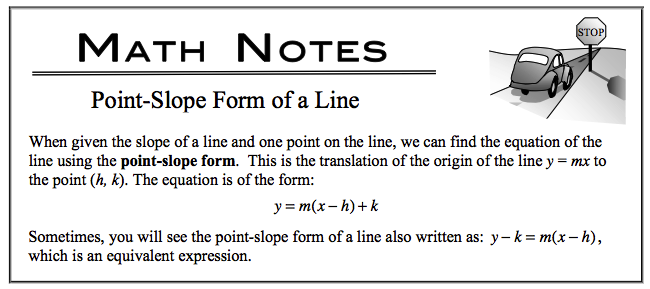### Home > CALC > Chapter 1 > Lesson 1.4.2 > Problem1-156

1-156.

Find the equation of the line parallel to $9y - 4x = 12$ through the point $(6, -7)$. Write the equation in point-slope form shown in the Math Notes box following problem 1-8.

Since parallel lines have the same slope, find the slope by rearranging the equation so that $y =$ ____________.

To convert to point-slope, see the notes below: